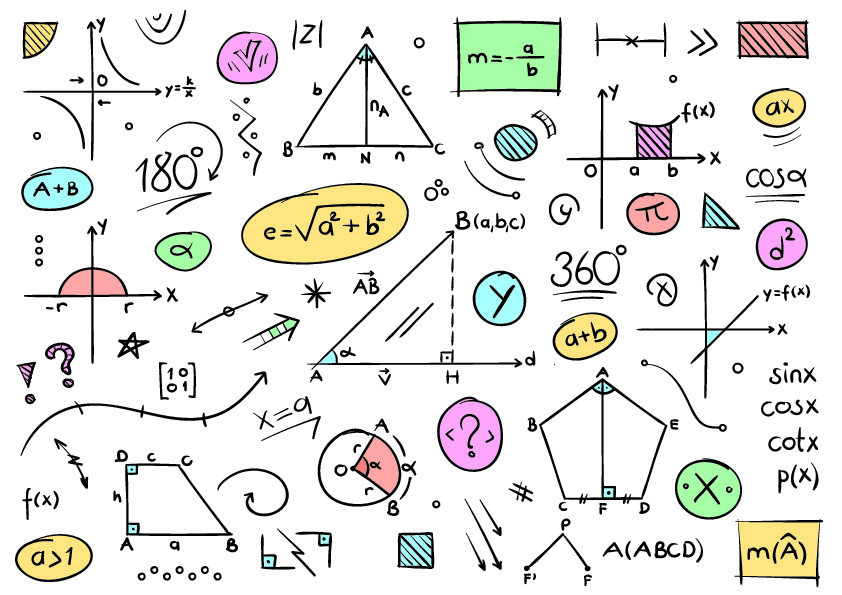# Geometry Questions: Right Triangle Magic

One of the first concepts students study in Geometry classes or tutoring sessions is typically a lesson on right triangles. While Geometry focuses on studying all different shapes and angles, right triangles are probably the easiest to learn. From there, teachers and tutors move on to additional, more complex types of angles and other shapes.# Geometry Questions: Right Triangle Magic

Last Updated on September 30, 2020 by A Plus In-Home Tutors

# One of the first concepts students study in Geometry classes or tutoring sessions is typically a lesson on right triangles. While Geometry focuses on studying all different shapes and angles, right triangles are probably the easiest to learn. From there, teachers and tutors move on to additional, more complex types of angles and other shapes.

##### What is a right triangle in Geometry?A right triangle is one that has a right angle, or a 90 degree angle.

How can I accurately measure the sides of a right triangle?
On your own or with the help of an excellent Geometry tutor, you can easily find the lengths of the sides of a right triangle. Simply, treat each side as if it was its own square. Find the area of the two sides of the triangle that make up the right angle. From there, you can calculate the other side (hypotenuse).
The length of A squared + the length of B squared = C squared (the hypotenuse).

I keep hearing my Geometry teacher and tutor talk about the Pythagorean Theorem. What is that?
In Geometry, The Pythagorean Theorem is the theorem just mentioned above about how to measure the length of the hypotenuse.
(Length of A squared + length of B squared = length of C squared)

Can a right triangle also be any other type of triangle in Geometry?
Yes, a right triangle can also be two different types of triangles.
First, a right triangle can also be an isosceles triangle. This happens often in the study of Geometry when one angle is 90 degrees, and the other two are both 45 degrees.
Second, a right triangle can also be a 30-60-90 triangle. This happens less often in Geometry, but occurs when the angles of the triangle are all different. One is 30 degrees, one is 60 degrees, and then there is the 90 degree angle thus making it a perfect right triangle.

Where else can I go for help with right triangles?
Right triangles are one of the stepping stones in a basic Geometry class. If you need help understanding the concept, you should consult with your teacher or get the help of a Tustin tutor. When you master understanding the Geometry concepts associated with right triangles, then you’ll probably understand the rest of Geometry with ease. Whether you live in Tustin, Huntington Beach, or Fullerton, it is very important to seek help early on in Geometry before you start to experience too much difficulty.

www.APlusInHomeTutors.com